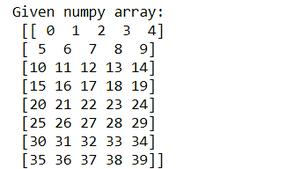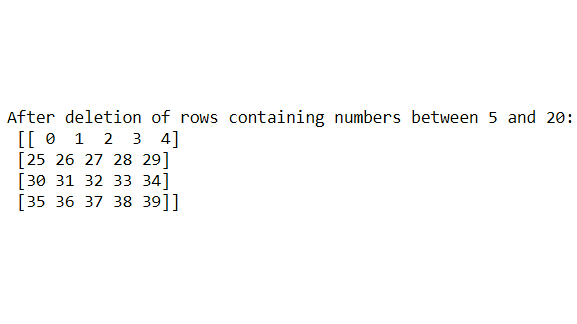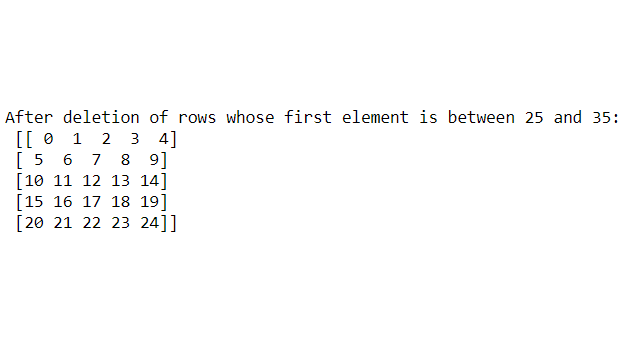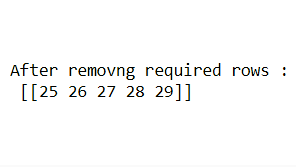# How to remove rows from a Numpy array based on multiple conditions ?

In this article, we will learn how to remove rows from a NumPy array based on multiple conditions. For doing our task, we will need some inbuilt methods provided by the NumPy module which are as follows:

• np.delete(ndarray, index, axis): Delete items of rows or columns from the NumPy array based on given index conditions and axis specified, the parameter ndarray is the array on which the manipulation will happen, the index is the particular rows based on conditions to be deleted, axis=0 for removing rows in our case.
• np.where(conditions): Operate on array items depending on conditions on rows or columns depending on the axis given.

Note: For 2-dimensional NumPy arrays, rows are removed if axis=0, and columns are removed if axis=1. But here we intend is to remove rows, so we will keep axis=0.

Let us take the NumPy array sample. Here we have taken a NumPy array having elements from  0 to 40 and reshaped the array into 8 rows and 5 columns.

## Python3

 `import` `numpy as np`   `nparray ``=` `np.arange(``40``).reshape((``8``, ``5``))` `print``(``"Given numpy array:\n"``, nparray)`

Output:Example 1: Remove rows having elements between 5 and 20 from the NumPy array

Here np.where((nparray >= 5) & (nparray <= 20)), axis=0) means it will delete the rows in which there is at least one or more elements that is greater than or equal to 5 and less than or equal to 20. So, 2nd, 3rd,4th, and 5th rows have elements according to the conditions given, so it gets deleted or removed.

## Python3

 `nparray ``=` `np.delete(nparray, np.where(` `    ``(nparray >``=` `5``) & (nparray <``=` `20``))[``0``], axis``=``0``)`   `print``("After deletion of rows containing` `      ``numbers between ``5` `and` `20``: \n", nparray)`

Output:Example 2: Remove rows whose first element is greater than 25 and less than 35 from the NumPy array

Here (np.where(nparray[:, 0] >= 25) & (nparray[:, 0] <= 35)), axis=0)means it will delete the rows in which there is at least one or more elements whose first element is greater than or equal to 25 and less than to equal to 35. The nparray[:, 0] to point to the first element of each row. So, 6th, 7th, 8th rows have elements according to the conditions given, so it gets deleted or removed.

## Python3

 `nparray ``=` `np.delete(nparray, np.where(` `    ``(nparray[:, ``0``] >``=` `25``) & (nparray[:, ``0``] <``=` `35``))[``0``], axis``=``0``)`   `print``("After deletion of rows whose first element \` `is` `between ``25` `and` `35``:\n", nparray)`

Output:Example 3: Remove rows whose third item is divisible by 2, 5th and 4th items are divisible by 3

Here np.where((nparray[:, 2] % 2 == 0) | (nparray[:, 4] % 3 == 0)| (nparray[:, 3] % 3 == 0)), axis=0) means it will delete the rows in which there is at least one or more elements whose 3rd column item(s) are divisible by 3 or rows in which there is at least one or more elements whose 5th and 4th column item(s) are divisible by 3 axis=0 to remove the rows. The nparray[:, 2], nparray[:, 4], nparray[:, 3] to point to the third, 5th, and 4th item of each row respectively. So, 6th, 7rd, 8th rows have elements according to the conditions given, so it gets deleted or removed.

## Python3

 `nparray ``=` `np.delete(nparray, np.where((nparray[:, ``2``] ``%` `2` `=``=` `0``) | (` `    ``nparray[:, ``4``] ``%` `3` `=``=` `0``) | (nparray[:, ``3``] ``%` `3` `=``=` `0``))[``0``], axis``=``0``)`   `print``(``"After removing required rows :\n"``, nparray)`

Output:Whether you're preparing for your first job interview or aiming to upskill in this ever-evolving tech landscape, GeeksforGeeks Courses are your key to success. We provide top-quality content at affordable prices, all geared towards accelerating your growth in a time-bound manner. Join the millions we've already empowered, and we're here to do the same for you. Don't miss out - check it out now!

Previous
Next